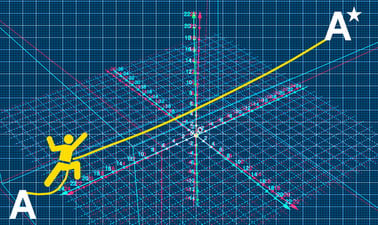# A-level Further Mathematics for Year 12 - Course 1: Complex Numbers, Matrices, Roots of Polynomial Equations and Vectors

Develop your thinking skills, fluency and confidence in A-level further maths and prepare for undergraduate STEM degrees.### There is one session available:

After a course session ends, it will be archived.
Started Jan 11
Ends Jan 31, 2023
Estimated 7 weeks
2–4 hours per week
Instructor-paced
Instructor-led on a course schedule
Free

This course by Imperial College London is designed to help you develop the skills you need to succeed in your A-level further maths exams.

You will investigate key topic areas to gain a deeper understanding of the skills and techniques that you can apply throughout your A-level study. These skills include:

• Fluency – selecting and applying correct methods to answer with speed and efficiency
• Confidence – critically assessing mathematical methods and investigating ways to apply them
• Problem-solving – analysing the ‘unfamiliar’ and identifying which skills and techniques you require to answer questions
• Constructing mathematical argument – using mathematical tools such as diagrams, graphs, logical deduction, mathematical symbols, mathematical language, construct mathematical argument and present precisely to others
• Deep reasoning – analysing and critiquing mathematical techniques, arguments, formulae and proofs to comprehend how they can be applied

Over eight modules, you will be introduced to

• complex numbers, their modulus and argument and how they can be represented diagrammatically
• matrices, their order, determinant and inverse and their application to linear transformation
• roots of polynomial equations and their relationship to coefficients
• series, partial fractions and the method of differences
• vectors, their scalar produce and how they can be used to define straight lines and planes in 2 and 3 dimensions.

Your initial skillset will be extended to give a clear understanding of how background knowledge underpins the A-level further mathematics course. You’ll also be encouraged to consider how what you know fits into the wider mathematical world.

### At a glance

• Institution: ImperialX
• Subject: Math
• Level: Intermediate
• Prerequisites:

N/A

• Language: English
• Video Transcript: English

# What you'll learn

Skip What you'll learn

How to extend the number system to includeand the definition of a complex number.

How to add, subtract, multiply and divide complex numbers.

How to represent complex numbers on an Argand diagram and the modulus and argument of a complex number.

How to write complex numbers in modulus-argument form.

How to define loci in the complex plane.

How to define a matrix by its order.

How to add and subtract conformable matrices.

How to multiply two conformable matrices.

How to use matrices to define linear transformations.

How to find invariant lines and lines of invariant points.

How to find the determinant and inverse of a 2 x 2 and 3 x 3 matrix.

How to use matrices to solve systems of linear equations.

How to use standard series formulae to find the sums of other series.

How to separate algebraic fractions into partial fractions.

How to use the method of differences to find the sum of a series.

How to find the scalar (dot) product of two vectors.

How to define the equation of a line using vectors.

How to define a plane using vectors.

How to use vectors to solve problems involving lines and planes.

# Syllabus

Skip Syllabus

Module 1: Complex Numbers 1: An Introduction to Complex Numbers

• The definition of an imaginary number
• The definition of a complex number
• Addition, subtraction and multiplication of complex numbers
• Complex conjugates and division of complex numbers
• Representing complex numbers on the Argand diagram

Module 2: Matrices 1: An Introduction to Matrices

• The order of a matrix
• Addition and subtraction of conformable matrices
• Matrix multiplication
• The identity matrix
• Matrix transformations in 2 and 3 dimensions
• Invariant lines and lines of invariant points

Module 3: Further Algebra and Functions 1: Roots of Polynomial Equations

• Solving polynomial equations with real coefficients
• The relationship between roots and coefficients in a polynomial equation
• Forming a polynomial equation whose roots are a linear transformation of the roots of another polynomial equation

Module 4: Complex Numbers 2: Modulus-Argument form and Loci

• The modulus and argument of a complex number
• Writing complex numbers in modulus argument form
• The geometrical effect of multiplying by a complex number.

• Loci on the Argand diagram

Module 5: Matrices 2: Determinants and Inverse Matrices

• The determinant of asquare matrix.
• The inverse of asquare matrix
• Using matrices to solve simultaneous equations (5)
• The geometrical interpretation of the solution of a system of equations

Module 6: Further Algebra and Functions 2: Series, Partial Fractions and the Method of Differences

• Deriving formulae for series using standard formulae
• Separating algebraic fractions into partial fractions
• The method of differences
• Partial fractions and method of differences

Module 7: Vectors 1: The Scalar (dot) Product and Vector Equations of Lines

• The scalar product of two vectors
• The vector and Cartesian forms of an equation of a straight line in 2 and 3 dimensions
• Solving geometrical problems using vector equations of lines
• The dot product and the angle between two lines

Module 8: Vectors 2: The Vector Equations of a Plane and Geometrical Problems with Lines and Planes

• The vector and Cartesian forms of the equation of a plane
• The vector equation of a plane
• Solving geometrical problems with lines and planes using vectors
• The intersection of a line and a plane
• Perpendicular distance from a point to a plane

# Learner testimonials

Skip Learner testimonials

N/A